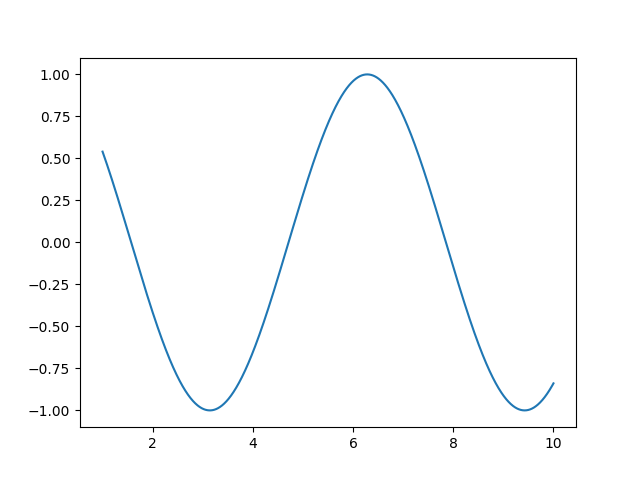# 9.1.1. Basic numerics and plotting with Python¶

A simple example of basic Python numerics and how to plot it.Out:

```[<matplotlib.lines.Line2D object at 0x7fa52dde7fd0>]
```

```# import numpy: the module providing numerical arrays
import numpy as np
t = np.linspace(1, 10, 2000)

# import matplotlib.pyplot: the module for scientific plotting
import matplotlib.pyplot as plt
plt.plot(t, np.cos(t))
```

Total running time of the script: ( 0 minutes 0.165 seconds)

Estimated memory usage: 9 MB

Gallery generated by Sphinx-Gallery